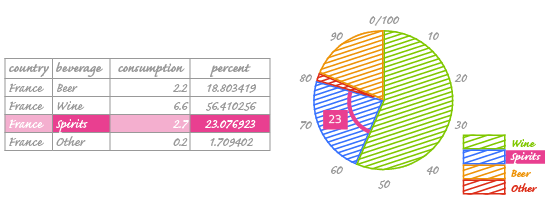Deals Of The Week - hours only!Up to 80% off on all courses and bundles.-Close
Introduction
Visualize your data - pie charts
10. How data is encoded in a pie chart
Check yourself

## Instruction

In the previous exercise, we prepared data for our chart. Let's look again at how data is encoded in a pie chart:We can see that the name of the beverage (Spirits) is in the legend on the right side of the pie. The percentage (23) corresponds to the size and angle of the wedge.

Wedge angles are the most important encoding on a pie chart. We can use angles to encode data because of the polar coordinate system. This uses angles and the radius of a circle as coordinates. For a pie chart, we fix the radius and focus on the angle. Readers can determine the angle by using the straight lines radiating from the center of the circle to its edge. This gives us the familiar wedge shape.

Changing the classic perpendicular coordinate system (like we use on bar charts and histograms) to the polar system involves converting percentages to angles. In other words, we change the numbers from a 0-100 percent range to a 0-360 degree range. We use the following formula to do this:

angle = percent / 100 × 360

So if we have a value of 23 percent, we can calculate its degrees like this:

23 / 100 × 360 = 82.8 degrees

This value is shown on the pie chart. As you can see, it's not as complicated as it seems. We can visualize percentages as parts of a whole circle, which helps us grasp the problem of presenting part of a whole.

Now we know how angles work in pie charts. However, wedge angles are not the most effective encoding technique; users can have problems correctly interpreting the area of the wedges. We'll discuss how to fix this later.

Although we discussed the math involved in converting percentages to degrees on a circle, ggplot will do the conversion for you as it plots the chart.